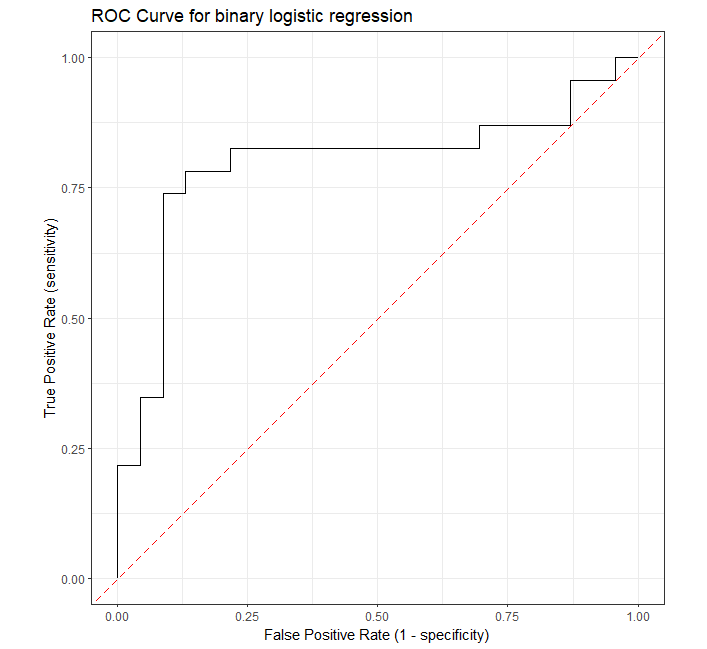# How to Plot ROC Curve in R

The Receiver Operating Characteristic (ROC) curve is a graphical plot for evaluating the performance of binary classification models such as logistic regression, support vector machines, etc.

ROC curve visualizes the trade-off between sensitivity (true positive rate) and specificity (false positive rate) for all possible threshold values.

A model with good predictability will have ROC curve that extends towards the upper-left corner of the plot (high true positive rate and low false positive rate). A perfect prediction model will have an ROC curve with `true positive rate (TPR)` = 1 and `false positive rate (FPR)` = 0.

In R, the ROC curve can be plotted using the `roc_curve()` function from the `yardstick` package.

Let’s take the example of the logistic regression to plot the ROC curve in R

Fit the logistic regression model using the sample breast cancer dataset. This dataset contains the four features and the response (whether the patient is cancerous or healthy).

``````# load data

# view first few rows
# diagnosis is a target variable with two levels with cancer (1) or healthy (0) patients
Age      BMI Glucose Insulin diagnosis
1  48 23.50000      70   2.707         0
2  83 20.69049      92   3.115         0

# fit logistic regression model
fit = glm(diagnosis ~ Age + BMI + Glucose + Insulin, family = binomial(), data = train_df)
``````

Perform the prediction on test dataset using the fitted model,

``````# load test dataset

# view first few rows
Age   BMI Glucose Insulin diagnosis
1  75 23.00      83   4.952         0
2  34 21.47      78   3.469         0

# perform prediction
pred_probs <- predict(fit, test_df, type = "response")
``````

Plot the ROC curve,

``````# load packages
library(yardstick)
library(ggplot2)
library(dplyr)

# create a data frame of truth value and predicted probabilities
roc_df <- data.frame(test_df\$diagnosis, pred_probs)
colnames(roc_df) <- c("truth", "pred_probs")
roc_df\$truth <- as.factor(roc_df\$truth)

# plot ROC
roc_curve(roc_df, truth, pred_probs, event_level = "second") %>%
ggplot(aes(x = 1 - specificity, y = sensitivity)) +
geom_path() +
geom_abline(lty = 5, col = "red") +
coord_equal() +
xlab("False Positive Rate (1 - specificity)") +
ylab("True Positive Rate (sensitivity)") +
ggtitle("ROC Curve for binary logistic regression") +
theme_bw()
``````

Note: In `roc_curve()`, the `event_level` describes the event of interest in the target variable (`diagnosis`). By default, it uses the first level as an event of interest.Related: Calculate AUC in R

## Subscribe to get new article to your email when published

* indicates required